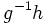# Proving product of subgroups

This is a survey article related to:product of subgroups
View other survey articles about product of subgroups

## Introduction

This article is about general strategies used to prove that a given group, is a product of two subgroups we have at hand. These techniques include situations where both subgroups are normal, where only one is normal, and where neither is normal.

## The group action method

Suppose we need to prove that a given group$G$ can be expressed as the product of two subgroups$H$ and$K$. The group action method is as follows. We find an action of the group$G$ on some set$S$ such that:

•$K$ contains the isotropy subgroup of some point$s \in S$
•$H$ acts transitively on$S$ (the action being given by restriction from$G$)

Under these circumstances, we can show that$G = HK$, as follows:

• Pick any$g \in G$
• Find a$k \in K$ such that$gs = hs$
• Thus,$g^{-1}h$ fixes$s$, so$h^{-1}g \in K$. We'll thus get$g = hk$ for some$k \in K$

Note that since$G = HK$ is equivalent to$G = KH$, we can construct a group action with the roles of$H$ and$K$ reversed.

Described below are some applications of this method.

### Frattini's argument

Further information: Frattini's argument

The setup here is as follows:$G$ is a group,$H$ is a normal subgroup, and$P$ is an automorph-conjugate subgroup of$H$. We need to show that:$HN_G(P) = G$

Here,$N_G(P)$ is the normalizer of$P$ in$G$.

The set$S$ here is the set of all conjugates of$P$ in$G$. The$G$-action is by conjugation.

• The isotropy at$P$ is$N_G(P)$: This is by definition of normalizer
• Since$H$ is normal in$G$, any conjugate to$P$ in$G$ is an automorph of$P$ in$H$. Moreover,$P$ was chosen to be automorph-conjugate, so any conjugate of$P$ in$G$ is actually conjugate to it in$H$. Hence,$H$ acts transitively on$S$.

### Finding the Weyl group

Further information: Computing the Weyl group of the unitary group

This is the idea used to prove that in the unitary group$U(n)$, the normalizer of any torus$T$ is given by:$N(T) = TS_n$

where$S_n$ is the symmetric group on$n$ letters, embedded as matrices inside$U(n)$.

Pick an element in$T$ with distinct diagonal entries. Elementary linear algebra tells us that any conjugate of it in$T$, must have the same diagonal entries, possibly in a different order. Thus, its$N(T)$-orbit is precisely the set of$n!$ different diagonal matrices with exactly those diagonal entries.

Let$N(T)$ act on this finite set, by conjugation.

• The isotropy of any point in the set is exactly$T$. This is based on the linear algebra fact that any matrix that commutes with a diagonal matrix with distinct diagonal entries, must also be diagonal.
• The symmetric group$S_n$, viewed as permutation matrices, acts transitively by conjugation.

## Another variant of the group action method

In this variant, instead of computing the isotropy at one point, we do the following: Find an action of$G$ on a set$S$, such that:

•$K$ contains the kernel of the action (i.e. any element that acts trivially on$S$, lives in$K$)
• For any element of$G$, there exists an element of$H$ that has exactly the same action on$S$

Somewhere in between this and the previous approach is an approach where we look at the intersection of isotropies of some elements of$S$.

### Equivalence of definitions of central factor

Further information: Equivalence of definitions of central factor

There are two definitions of central factor:

1.$H$ is a central factor of$G$ if$HC_G(H) = G$ (where$C_G(H)$ is the centralizer of$H$ in$G$)
2.$H$ is a central factor of$G$ if every inner automorphism of$G$ restricts to an inner automorphism of$H$

We'll show here that (2) implies (1):

The set$S$ is the set of elements of$H$. The$G$ action is by conjugation. This action is well-defined because (2) in particular indicates that$H$ is normal in$G$.

• The kernel of the action is precisely$C_G(H)$
• By condition (2), we have that for any element of$G$, there exists an element in$H$ such that the action by conjugation on$H$ is the same for both.

This gives the result.

A similar technique is used to prove the equivalence of definitions of WC-subgroup.